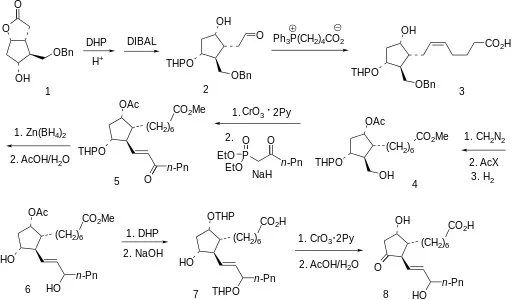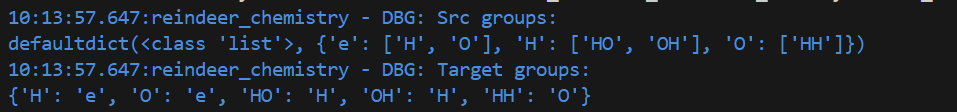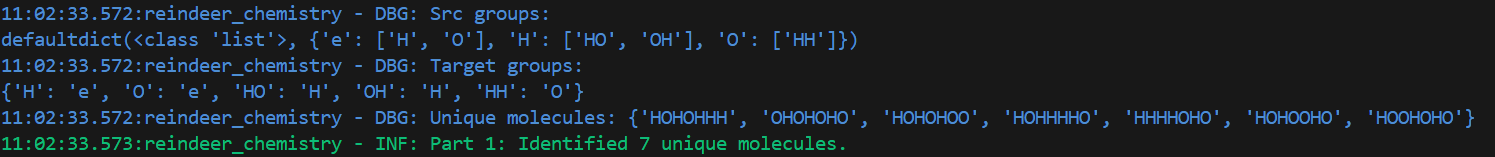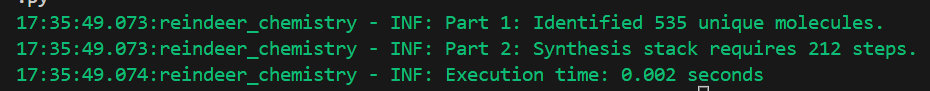# Learning Python with Advent of Code Walkthroughs

Dazbo's Advent of Code solutions, written in Python# Advent of Code 2015 - Day 19

## Problem Intro

We’re told Rudolph needs medicine, and we’re going to need to synthesize the medicine! We are able to construct molecules using reindeer chemistry. We’re told reindeer chemistry works by starting with some input molecule and then doing a series of replacements, one per step, until we have the right target molecule.

We’re given sample input that looks something like this:

``````e => H
e => O
H => HO
H => OH
O => HH

HOHOHO
``````

The last row is the the medicine molecule and the other rows are the possible replacements. Note how some molecules can be replaced with more than one target molecule.

## Part 1

First, we need to calibrate the reindeer chemistry machine.

How many distinct molecules can be created after all the different ways you can do one replacement on the medicine molecule?

I start by reading in the data and constructing a mapping dictionary from each source molecule to the target molecules, and for each target molecule to its source:

``````def process_input(data: list) -> tuple[dict, dict, str]:
subst_match = re.compile(r"^(\w+) => (\w+)")

# each src group can make many target groups
src_groups = defaultdict(list)

# each target group can be made from only one src group
target_groups = {}

for line in data:
if "=>" in line:
group, target_group = subst_match.findall(line)
src_groups[group] += [target_group]
target_groups[target_group] = group

logger.debug("Src groups:\n%s", src_groups)
logger.debug("Target groups:\n%s", target_groups)
return src_groups, target_groups, data[-1]
``````

I’m using regex to parse the input lines for each group (nothing new here), and then - for the source groups - I’m using a defaultdict. This is useful, because I want the `dict` key to be the source molecule, and the `dict` value to be a `list` of target molecules. But I don’t want to have to create the `list` for the first target molecule.

With the sample input above, this gives the following output:Now, to solve part 2, my strategy is:

• Iterate through through the `dict` of source groups. Recall that each dict item will be composed of a source group, and a list of of possible substitutions for that source group.
• For each source, look for all matches of this source group, in the medicine molecule.
• Every place there is a match, substitude (in turn) with each of the possible substitutions.

All of this is done in the `substitute_groups` function:

``````def substitute_groups(groups: dict, molecule: str) -> list:
new_molecules = []

# go through all the groups we have substitutions for
for group, targets in groups.items():
# get all matching positions for this group
group_matches = re.finditer(group, molecule)

# move left to right, matching group one at a time
for group_match in group_matches:
start, end = group_match.span()
prefix = molecule[:start]
suffix = molecule[end:]

# replace the current group occurrence with each target
for target in targets:
new_molecules.append(prefix + target + suffix)

return new_molecules
``````

It works by using `finditer()` to return matches for all the source groups. This is useful, because each match has a start and end position in the overall string. We can use these start and end positions to define the portion of the string that will be replaced, whilst leaving the rest of the string untouched. So, for each match, we construct a new target molecule, by replacing the source molecule with the next available target molecule.

So, we’re ready to solve Part 1:

``````    with open(locations.sample_input_file, mode="rt") as f:

src_groups, target_groups, medicine_molecule = process_input(data)

new_molecules = substitute_groups(src_groups, medicine_molecule)
unique_new_molecules = set(new_molecules)
logger.debug("Unique molecules: %s", unique_new_molecules)
logger.info("Part 1: Identified %d unique molecules.", len(unique_new_molecules))
``````

Note that I’m using a `set` to remove duplicate molecules.

Using the sample input, the resulting output looks like this:Good, that works!

## Part 2

Now we’re ready to fabricate our target molecule. We’re told we start with a single electron `e`, and apply replacements one a time. Each replacement counts as one step.

Given the available replacements and the medicine molecule in your puzzle input, what is the fewest number of steps to go from e to the medicine molecule?

Here’s my strategy:

• Start with the goal (medicine) molecule, e.g. `HOHOHO`.
• Determine which target groups our goal molecule is composed of. Do this using a non-overlapping search. For example, in our list of substitutions, we find we have the target_group `OH` three times. (Note that each target molecule can only be made from a single source molecule. Whereas a single source molecule can potentially be converted to more than one target molecule.)
• Perform the reverse substitution in every location, and make this our new goal molecule. For example, `OH` can only be made from `H`. So if we perform this reverse substitutioin, then `HOHOHO` becomes `HHH`.
• Append this new molecule, and the number of substitutions that were required to make it, to a stack.
I.e. we now have a `sythesis_stack` that contains `[[3, "HHH"]]`.
• Now repeat this loop.

Second time around (with the sample data):

• We start with `HHH`.
• This time, the only target molecule that is part of `HHH` is `HH`. With a non-overlapping count, we see that `HH` only occurs one in `HHH`.
• `HH` is made from `O`. So if we do the reverse substitution, then `HHH` becomes `OH`.
• Append this new molecule to the stack. So our `synthesis_stack` now looks like `[[3, "HHH"], [1, "OH"]]`:

Third time around:

• We start with `OH`.
• This contains just one instance of the target molecule `OH`, which is made from the source molecule `H`. So, perform this reverse substitution.
• Append the resulting new molecule to the stack: `[[3, "HHH"], [1, "OH"], [1, "H"]]`

Finally, we see that `H` can only be made from `e`. So, performing the final reverse substitution, we end up with the stack: `[[3, "HHH"], [1, "OH"], [1, "H"], [1, "e"]]`

If we reverse the stack, we can see that we assemble the target medicine molecule like this:

``````1: e -> H
2: H -> OH
3: (O)H -> (HH)H
4: (H)HH -> (HO)HH
5: HO(H)H -> HO(HO)H
6: HOHO(H) -> HOHO(HO)
``````

Here’s my code to achieve this:

``````def retrosynthesis(target_groups: dict, target_molecule: str) -> list:
synthesis_stack = []
current_molecule = target_molecule

# start by doing all the substitions, without e
# repeat until the molecule is not modified
molecule_modified = True
while molecule_modified:
molecule_modified = False

for tgt_grp, src_grp in target_groups.items():
if src_grp == 'e':
continue

# count how many matches of target first
substitutions = current_molecule.count(tgt_grp)

# then replace them all
if substitutions > 0:
current_molecule = current_molecule.replace(tgt_grp, src_grp)
molecule_modified = True
synthesis_stack.append([substitutions, current_molecule])

# now replace target with e
for tgt_grp, src_grp in target_groups.items():
if src_grp != 'e':
continue

# count how many matches of target first
substitutions = current_molecule.count(tgt_grp)

# then replace them all
if substitutions > 0:
current_molecule = current_molecule.replace(tgt_grp, src_grp)
synthesis_stack.append([substitutions, current_molecule])

return synthesis_stack
``````

And we can now solve Part 2 like this:

``````    synthesis_stack = retrosynthesis(target_groups, medicine_molecule)
synthesis_steps = sum(subs for subs, molecule in synthesis_stack)
logger.debug(synthesis_stack)
logger.info("Part 2: Synthesis stack requires %d steps.", synthesis_steps)
``````

Note the use of the `sum()` aggregate function on the dictionary comprehension, which is a nice Pythonic way of summing up all the substitutions.

## Results

If I set my logging level to `INFO` rather than `DEBUG`, and run with my real data, the output looks like this:So, it’s pretty quick!